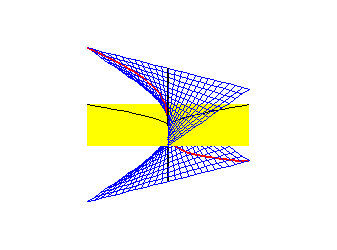# Introduction to Algebraic Geometry

Donu ArapuraBlow up of y2=x3

In a sentence, algebraic geometry is the study of solutions to algebraic equations. People learning it for the first time, would see a lot of algebra, but not much geometry. But it is there. The picture above depicts a resolution of the singular curve y2=x3. This can be accomplished by taking integral closures on the algebra side, or by doing a blow up. This curve drawn in black is resolved by blowing up the plane at the origin (replacing the yellow by blue surface) and taking the closure of the preimage away from the origin (in red).

For a really elementary introduction, go here.

For a more serious introduction, you can get my notes on basic algebraic geometry. This is sort of a "prequel" to Hartshorne. (It's a 340K PDF file)

My notes "Algebraic geometry over the complex numbers" covers more: sheaf theory, cohomology and Hodge theory. (It's a 1.3 MB PDF file).